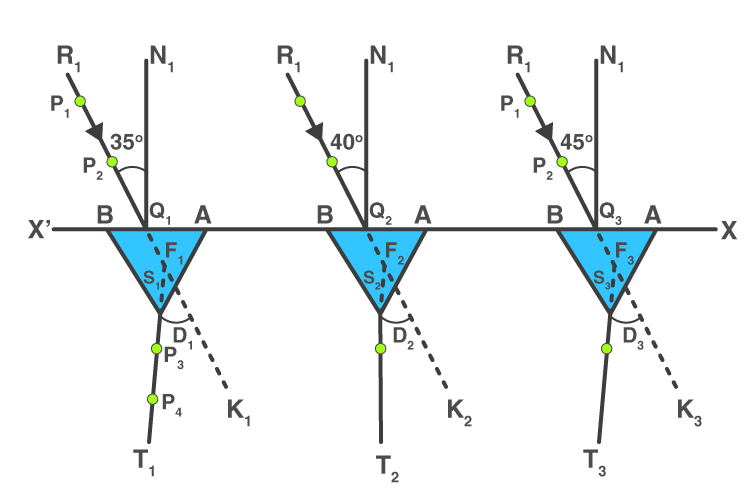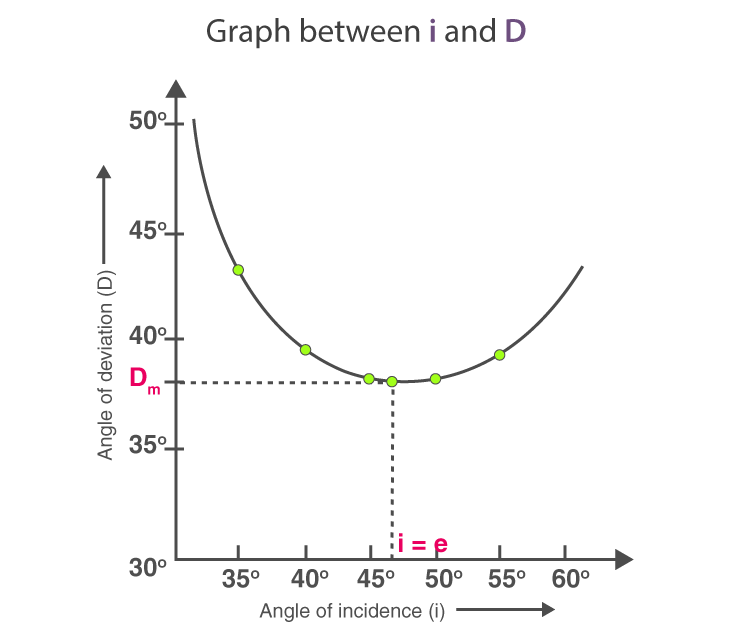# To determine angle of minimum deviation for a given prism by plotting a graph between angle of incidence and angle of deviation

## Aim

To determine the angle of minimum deviation for a given prism by plotting a graph between angle of incidence and angle of deviation.

## Materials Required

• A drawing board
• A white sheet of paper
• A prism
• Drawing pins
• Pencil
• A half-metre scale
• Office pins
• Protractor
• Graph paper

## Theory

Refraction occurs when a light ray travels from one medium to another resulting in deviation of the emergent ray from that of the incident ray. Following is the refractive index of the material used in the prism:

$$\begin{array}{l}n=\frac{sin(\frac{A+D_{m}}{2})}{sin(\frac{A}{2})}\end{array}$$

Where Dm is the angle of minimum deviation and A is the prism angle.

## Ray DiagramRefraction through prism at different angles

## Procedure

1. Place the white sheet of paper on the drawing board and fix it with the help of drawing pins.
2. XX’ is the straight line drawn parallel to the length of the paper such that it is in the middle of the paper.
3. Mark points Q1, Q2, Q3…… on the straight line XX’ at a distance of 5 cm each.
4. N1Q1, N2Q2,… are the normals that are drawn on the points Q1, Q2,… as shown in the ray diagram.
5. Make angles of 35°, 40°,….,60° by drawing straight lines R1Q1, R2Q2,…….. With respect to the normals.
6. To take one edge of the prism for all the observations, mark any corner of the prism as A.
7. Place the prism with its refracting face AB on the line XX’ and on the point Q1 and also in the middle of AB.
8. Mark the boundary of the prism.
9. On the line, R1Q1, fix office pins vertically and mark them as P1 and P2. the distance between these pins should not be less than 10mm.
10. Through the prism face, AC, look for the images of the points P1 and P2.
11. Close your left eye and with the right eye open, bring it in line with the two images.
12. Fix the other two office pins vertically and name them as P3 and P4. These pins should be 10cm apart from each other. P3 and P4 should be in one straight with respect to the images of P1 and P2.
13. Encircle the pricks of pins P3 and P4.
14. For points, Q2, Q3,…. for angle 40°, 45°,…..60°, repeat the steps 7 to 13.

To measure D in a different case

1. To get emergent rays S1T1, S2T2,….. draw straight lines through P4 and P5.
2. Inward the boundary of the prism to produce T1S1, T2,S2….. so that they meet incident rays R1Q1, R2Q2,…. at points F1, F2,…
3. To obtain the angle of deviation D1, D2,…. measure the angles K1F1S1, K2F2,S2,…….
4. Note these angles.

To measure angle A

1. To get angle A, measure the angle BAC in the boundary of the prism.
2. Record the observations.

## Observations

The angle of prism, A = 60°

 Serial no. Angle of incidence ∠i Angle of deviation ∠D 1 35° 43° 2 40° 39° 3 45° 38° 4 50° 38° 5 55° 39° 6 60° 42°

## Calculations

To plot the graph between the angle of incidence ∠i and the angle of deviation ∠D, take ∠i along the x-axis and ∠D along the y-axis. Minimum deviation Dm can be found from the graph which would corresponding to the lowest point in the graph.Let the value of angle of minimum deviation, Dm = 37°

Then,

$$\begin{array}{l}n=\frac{sin(\frac{A+D_{m}}{2})}{sin(\frac{A}{2})}\end{array}$$

Putting the A = 60° and Dm = 37° in the above equation, we get n = 1.5

## Result

• The angle of minimum deviation, Dm = 37°
• Refractive index of the material of the prism, n = 1.5
• The graph indicates as the angle of incidence increases, the angle of deviation first decreases to attain the minimum value of Dm and then again increases with the angle of incidence.

## Precautions

• 35°-60° is the angle of incidence that needs to be maintained.
• The placement of the pins must be vertical.
• The placement of two pins should be such that the distance is not more than 10mm.
• To represent incident and emergent rays, arrowheads must be marked.
• The angle of prism used should be the same for all the observations.

## Sources of Error

• The pricks made by the pins might be thick.
• Angles might go wrong while measuring them.

## Viva Voice

Q1. Name the factors on which the angle of deviation depends.

Ans: Following are the factors on which the angle of deviation depends:

• The angle of incidence
• The refracting angle of the prism
• Refractive index of the material used in the prism
• The wavelength of the light ray

Q2. Define angular deviation.

Ans: Angular deviation is defined as the ratio of the angular dispersion of the two colours to produce the deviation by the prism.

Q3. Name the factor on which dispersive power depends.

Ans: Dispersive power depends on the refractive index of the material used in the prism.

Q4. Name the colours with the highest and smallest refractive index.

Ans: Violet colour has the highest refractive index while the red colour has the smallest refractive index.

Stay tuned with BYJU’S to learn more about other Physics experiments and other related concepts.

Test Your Knowledge On To Determine Minimum Deviation For Given Prism By Plotting Graph Between Angle Of Incidence And Angle Of Deviation!